# Length - problems

1. Photo frameA square shape photo with a side length of 20 cm is framed by a 4 cm wide bar. Find the external frame size of this photo (the frame is from all sides)
2. Tourist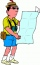A tourist walked an average speed of 3.5 km/h route in 6 hours. Calculate how many hours he would have passed at an average speed of 5.5km/h.
3. Geometric plan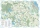At what scale the building plan if one side of the building is 45m long and 12mm long on a plan?
4. Cube into cylinderIf we dip a wooden cube into a barrel with a 40cm radius, the water will rise 10 cm. What is the size of the cube edge?
5. Medians 2:1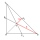Median to side b (tb) in triangle ABC is 12 cm long. a. What is the distance of the center of gravity T from the vertex B? b, Find the distance between T and the side b.
6. Iron pole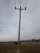The iron pole is in the ground 2/5 of its length, partly above the ground 1/3 is yellow, and the unpainted section is 6 m long. How long is the entire column?
7. Cyclist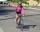Cyclist drives 5 km in half an hour, how long will drive in 3 hours?
8. Rocket start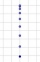The body launched vertically up returns to the start site in 6 seconds. What height did it have?
9. Sand castle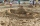Tim and Tom built a sand castle and embellished it with a flag. Half the pole with the flag plunged into the castle. The highest point of the pole was 80 cm above the ground, its lowest point 20 cm above the ground. How high was the sand castle?
10. LighthouseMarcel (point J) lies in the grass and sees the top of the tent (point T) and behind it the top of the lighthouse (P). | TT '| = 1.2m, | PP '| = 36m, | JT '| = 5m. Marcel lies 15 meters away from the sea (M). Calculate the lighthouse distance from the sea.
11. Athletic competitionIn a 400 meter athletic competition, a participant covers the distance as given below. find the average speed? first 80 meters 10 m/s next 240 meters 7.5 m/s last 80 meters 10 m/s
12. Cable carFind the elevation difference of the cable car when it rises by 67 per mille and the rope length is 930 m.
13. MG=7x-15,MG=7x-15, FG=33, x=? Point M is the midpoint of FG. Find unknown x.
14. Midpoint 6FM=8a+1, FG=42, a=? Point M is the midpoint of FG. Find unknown a.
15. Midpoint 5FM=3x-4, MG=5x-26, FG=? Point M is the midpoint of FG. Use the given information to find the missing measure or value.
16. GardenArea of square garden is 6/4 of triangle garden with sides 56 m, 35 m and 35 m. How many meters of fencing need to fence a square garden?
17. Right ΔRight triangle has length of one leg 28 cm and length of the hypotenuse 53 cm. Calculate the height of the triangle.
18. TroopsRoute is long 147 km and the first day first regiment went at an average speed 12 km/h and journey back 21 km/h. The second day went second regiment same route at an average speed 22 km/h there and back. Which regiment will take route longer?
19. Motion problemFrom Levíc to Košíc go car at speed 81 km/h. From Košíc to Levíc go another car at speed 69 km/h. How many minutes before the meeting will be cars 27 km away?
20. Cyclist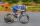A cyclist passes 88 km in 4 hours. How many kilometers he pass in 8 hours?

Do you have an interesting mathematical problem that you can't solve it? Enter it, and we can try to solve it.

To this e-mail address, we will reply solution; solved examples are also published here. Please enter e-mail correctly and check whether you don't have a full mailbox.

Do you want to convert length units?